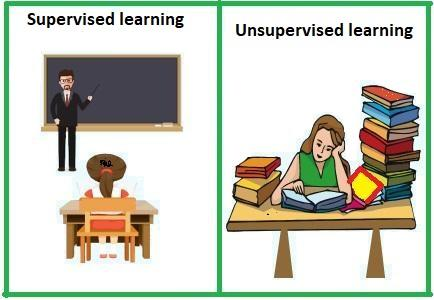# Supervised vs Unsupervised Machine Learning

In this page, we will learn about Supervised vs Unsupervised Machine Learning, What is the difference between Supervised and Unsupervised Learning?

## Supervised vs Unsupervised Machine Learning

Machine learning approaches include supervised and unsupervised learning. However, both strategies are employed in various contexts and with various datasets. Below is a description of two learning methods, as well as a comparison table.## Supervised Machine Learning:

Supervised learning is a machine learning technique that involves training models with labeled data. Models in supervised learning must discover a mapping function to connect the input variable (X) to the output variable (Y).

## Machine learning that is supervised

To train the model, supervised learning requires supervision, similar to how a student learns in the presence of a teacher. There are two sorts of issues that can be solved with supervised learning: classification and regression.

### Y = f(X)

Example: we have an image of many sorts of fruits. Our supervised learning model's job is to recognize the fruits and classify them appropriately. So, in order to recognize a picture in supervised learning, we'll provide both input and output data, which means we'll train the model based on the form, size, color, and taste of each fruit. After the training, we'll put the model to the test by feeding it a new batch of fruits. The model will recognize the fruit and, using a suitable algorithm, predict the outcome.

## Unsupervised Machine Learning:

Unsupervised learning is another machine learning method that uses unlabeled input data to discover patterns. Unsupervised learning aims to extract structure and patterns from unstructured data. There is no need for monitoring when learning unsupervised. Instead, it searches the data for patterns on its own. Clustering and Association are two sorts of issues that can be solved using unsupervised learning.

We will use the example presented above to better understand unsupervised learning. In contrast to supervised learning, we shall not give any supervision to the model in this case. We will simply feed the model with the input dataset and let it detect patterns in the data. With the help of a suitable algorithm, the model will train itself and divide the fruits into different groups according to the most similar features between them.

## What is the difference between Supervised and Unsupervised Learning?

Supervised Learning Unsupervised Learning
Labeled data is used to train supervised learning algorithms. Unsupervised learning algorithms are taught on data that hasn't been labeled.
The supervised learning model uses direct feedback to determine whether it is correctly anticipating output. There is no feedback in the unsupervised learning paradigm.
The outcome is predicted by a supervised learning model. The data is analyzed using an unsupervised learning model to uncover hidden patterns.
In supervised learning, the model receives both input and output data. Only input data is presented to the model in unsupervised learning.
The purpose of supervised learning is to train the model to predict the outcome when new data is provided. Unsupervised learning aims to uncover hidden patterns and meaningful insights in an unknown dataset.
To train the model, supervised learning is required. To train the model, unsupervised learning does not require any supervision.
Classification and regression issues are two types of supervised learning tasks. Clustering and Associations issues are two types of unsupervised learning tasks.
When we know both the input and the outputs, we can apply supervised learning. When there is only input data and no corresponding output data, unsupervised learning can be applied.
The supervised learning model yields a precise output. When compared to supervised learning, the unsupervised learning model may produce less accurate results.
Supervised learning is nowhere near to actual Artificial Intelligence because it requires us to train the model for each piece of data before it can anticipate the correct outcome. Unsupervised learning is closer to actual Artificial Intelligence since it learns in the same way that a toddler learns from his experiences.
Linear Regression, Logistic Regression, Support Vector Machine, Multi-class Classification, Decision Tree, Bayesian Logic, and more algorithms are included. Clustering, KNN, and the Apriori algorithm are among the algorithms included.

[Note: Both supervised and unsupervised learning are machine learning approaches, and the choice between the two depends on criteria such as the structure and volume of your dataset, as well as the problem's use cases.]